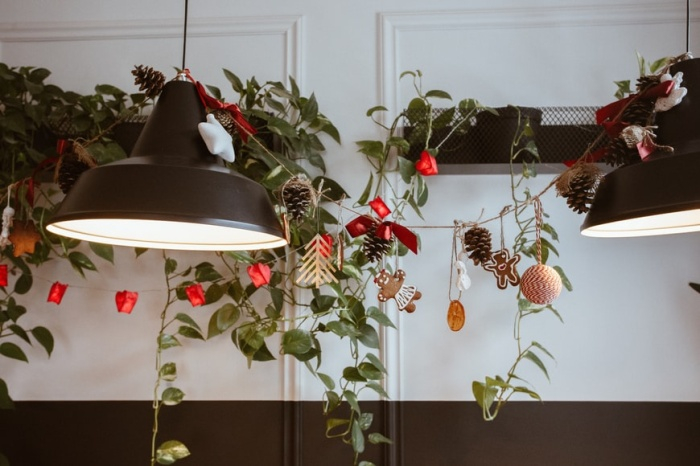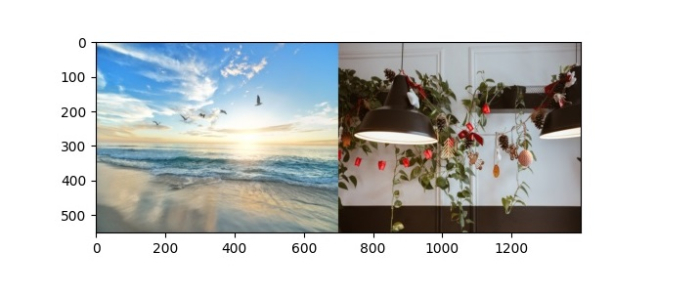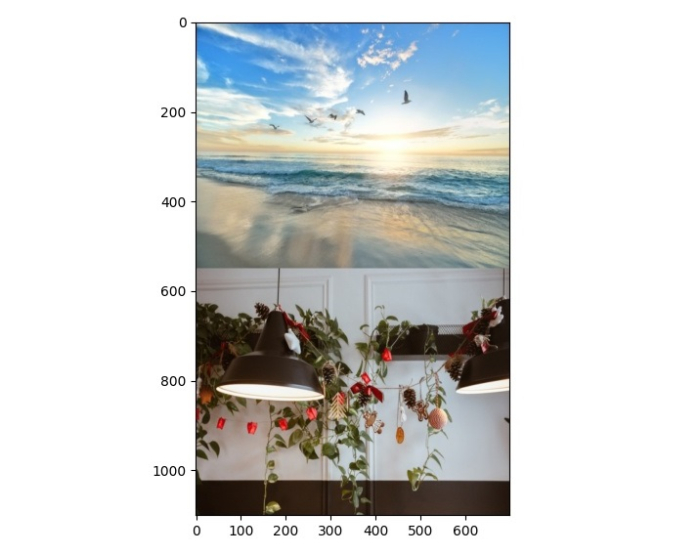# How to join two images horizontally and vertically using OpenCV Python?

Images in OpenCV are represented as numpy.ndarray. OpenCV provides two functions − cv2.hconcat() and cv2.vconcat() to join images.

The function cv2.hconcat() joins images horizontally and the function cv2.vconcat() joins images vertically.

• These functions join two or more images.

• These functions accept a list of images of the same size to join them.

• The height, width and number of channels of all images must be the same to join them

## Syntax

cv2.hconcat(img_list)
cv2.vconcat(img_list)


Where img_list is a list of images [img1, img2, …].

To join the images horizontally or vertically, one could follow the steps given below −

## Steps

Import the required libraries. In all the following Python examples, the required Python libraries are OpenCV and Matplotlib. Make sure you have already installed them.

import cv2
import matplotlib.pyplot as plt


Read the images using cv2.imread() function. Specify the full image path with image types (.jpg or .png).

img1 = cv2.imread('birds.jpg')


Resize the images if the size of images is not the same. The images of the same size can only be joined.

Join the images horizontally or vertically using cv2.hcocat() or cv2.vconcat() respectively.

img = cv2.hconcat([img1, img2])


Display the joined images

plt.imshow(img)


## Input Images

We will use these images as the input files in the following examples## Example 1

In the Python program below, we will join two images horizontally.

# import required library
import cv2
import matplotlib.pyplot as plt

# both image height and width should be same
img1 = cv2.resize(img1, (700, 550))
img2 = cv2.resize(img2, (700, 550))

# join the two images horizontally
img = cv2.hconcat([img1, img2])

# Convert the BGR image to RGB
img = cv2.cvtColor(img, cv2.COLOR_BGR2RGB)
plt.imshow(img)
plt.show()


## Output

When you run the above program, it will produce the following output window.## Example 2

The following program shows how you can join two images vertically.

# import required library
import cv2
import matplotlib.pyplot as plt

# both image height and width should be same
img1 = cv2.resize(img1, (700, 550))
img2 = cv2.resize(img2, (700, 550))

# join the two images vertically
img = cv2.vconcat([img1, img2])

# Convert the BGR image to RGB
img = cv2.cvtColor(img, cv2.COLOR_BGR2RGB)
plt.imshow(img)
plt.show()


## Output

When you run the above program, it will produce the following output window.